# Circles

A circle is the locus of a point which moves in such a way that its distance from a fixed point is always constant.

Circles## 1. The equation of a circle when the centre and radius are given.

Let C(h, k) be the centre and ‘r’ be the radius of the circle
Let P(x, y) be any point on the circle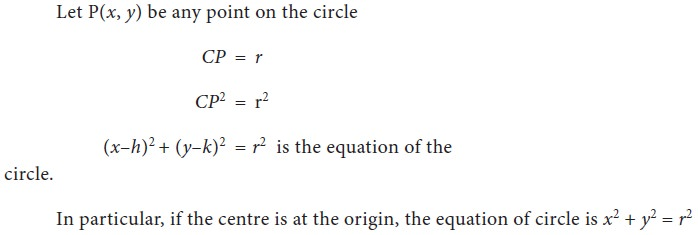## 2. Equation of a circle when the end points of a diameter are given## 3. General equation of a circle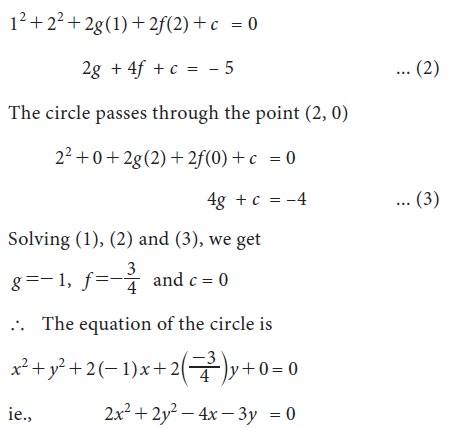## 4. Parametric form of a circle

Consider a circle with radius r and centre at the origin. Let P (x, y) be any point on the circle. Assume that OP makes an angle iwith the positive direction of x-axis.## 5. Tangents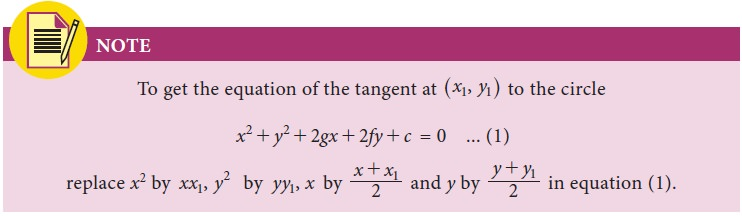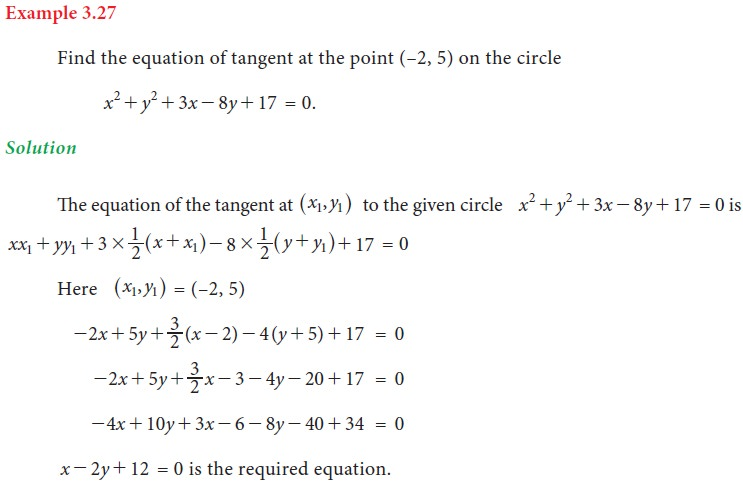### Length of the tangent to the circle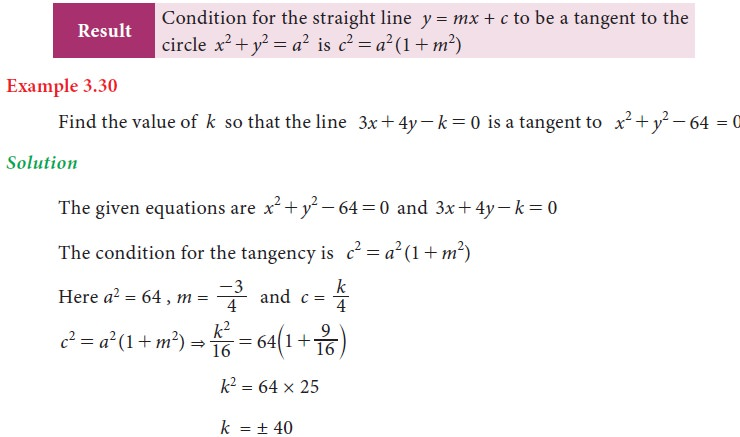Tags : Definition, equation, Formula, Solved Example Problems, Exercise | Mathematics , 11th Business Mathematics and Statistics(EMS) : Chapter 3 : Analytical Geometry
Study Material, Lecturing Notes, Assignment, Reference, Wiki description explanation, brief detail
11th Business Mathematics and Statistics(EMS) : Chapter 3 : Analytical Geometry : Circles | Definition, equation, Formula, Solved Example Problems, Exercise | Mathematics